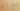OutsideInFunctionNesting

Misconception:

When nesting function calls, like with a(b()), calls are invoked from the outside in: first a() is invoked, then b() is invoked.Incorrect

Nested function calls are invoked outside in

Correct

Nested function calls are invoked inside out

CorrectionHere is what's right.

The correct way to execute nested function calls is inside out. For example, when evaluating the expression a(b()), first b() is called, producing a value, then a() is called, with the return value of b() as an argument.

Note that a will be evaluted (to a function object), but not called, before b() is called.

The corresponding expression tree looks as follows, assuming that b() returns a value of type T.

The fact that the call a() needs the return value of b() as an argument implies that b() has to be called before a(), and thus that these calls evaluate from the inside out.

SymptomsHow do you know your students might have this misconception?

This misconception occurred quite regularly, even for strong students. Here are two diagnostic probes to detect it.

Expression Tree

Ask students to draw a tree for the expression a(b()). Students with this misconception may draw the following incorrect tree, where the inner piece of the expression —b() — is drawn as the root:

Call Trace

Ask students to produce a call trace for code including the following statement:

compute(left.eval(), right.eval())

Students with this misconception may produce the following incorrect trace:

CALL compute
RETURN compute
CALL eval
RETURN eval
CALL eval
RETURN eval

Python